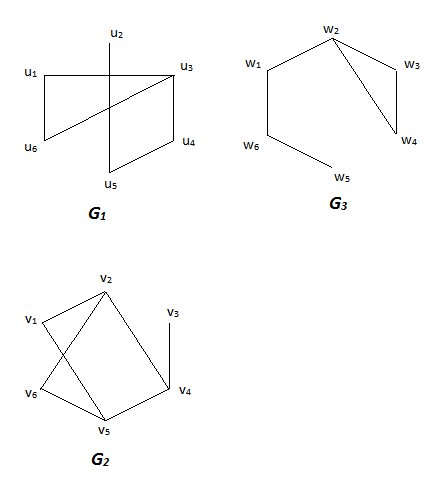# Determine whether any two of the simple graphs G_{1}, G_{2}, and G_{3} are isomorphic. If they...

## Question:

Determine whether any two of the simple graphs {eq}G_{1}, \;G_{2} {/eq}, and {eq}G_{3} {/eq} are isomorphic. If they are, give a vertex function that defines the isomorphism. If they are not, give an isomorphic invariant that they do not share.## Graphs and Graph Isomorphisms:

A graph is an ordered pair of sets {eq}G=(V,E) {/eq}, where each element of {eq}E {/eq} is a two-element subset {eq}\{v,w\} {/eq} of {eq}V {/eq}. The elements of {eq}V {/eq} are called the vertices of {eq}G {/eq}, while the elements of {eq}E {/eq} are called the edges of {eq}E {/eq}. Intuitively, graphs usually are used represent connectivity. That is, the vertices of a graph represent nodes in a network, and an edge between {eq}v {/eq} and {eq}w {/eq} denotes the fact that {eq}v {/eq} and {eq}w {/eq} are directly connected in that network.

Suppose that {eq}G_1=(V_1,E_1) {/eq} and {eq}G_2=(V_2,E_2) {/eq} are two graphs. An isomorphism between {eq}G_1 {/eq} and {eq}G_2 {/eq} is a function {eq}f:V_1 \to V_2 {/eq} such that:

1) {eq}f {/eq} is a bijection, and

2) For any {eq}v,w\in V_1 {/eq}, the set {eq}\{f(v),f(w)\} {/eq} is an element of {eq}E_2 {/eq} (an edge in {eq}G_2 {/eq}) if and only if the set {eq}\{v,w\} {/eq} is an element of {eq}E_1 {/eq} (an edge in {eq}G_1 {/eq}).

Two graphs {eq}G_1 {/eq} and {eq}G_2 {/eq} which have an isomorphism are said to be isomorphic. Intuitively, isomorphic graphs represent the same network structure.

## Answer and Explanation:

The graphs {eq}G_1 {/eq} and {eq}G_3 {/eq} are isomorphic. Intuitively, each of these graphs consists of a path of length 3 with a 3-cycle attached to one end, so they have the same structure. More formally, a vertex function defining the isomorphism is:

{eq}\begin{align*} u_1 &\to w_3\\ u_2 &\to w_5\\ u_3 &\to w_2\\ u_4 &\to w_1\\ u_5 &\to w_6\\ u_6 &\to w_4 \, . \end{align*} {/eq}

The graph {eq}G_2 {/eq} is not isomorphic to the other two. To see this, note that {eq}G_2 {/eq} has three vertices of degree 3 (the vertices {eq}v_2,v_4,v_5 {/eq}) while the other two graphs each only have one vertex of degree 3 (the vertices {eq}u_3 {/eq} and {eq}w_2 {/eq}). Since the number of vertices of a given degree is invariant under isomorphism, it follows that {eq}G_2 {/eq} is not isomorphic to {eq}G_1 {/eq} and {eq}G_3 {/eq}.

#### Learn more about this topic:Graph Theory Concepts and Terminology

from Math 106: Contemporary Math

Chapter 9 / Lesson 2
3.5K